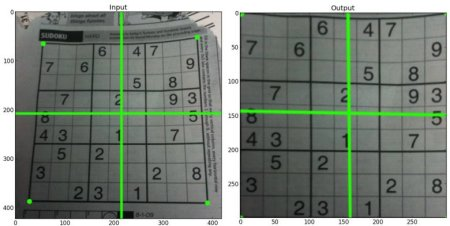# 图像的几何变换¶

### 目标¶

• 学习将不同的几何变换应用到图像上，如平移、旋转、仿射变换等。
• 你会看到这些函数: cv.getPerspectiveTransform

### 变换¶

OpenCV提供了两个转换函数**cv.warpAffine**和**cv.warpPerspective**，您可以使用它们进行各种转换。**cv.warpAffine**采用2x3转换矩阵，而**cv.warpPerspective**采用3x3转换矩阵作为输入。

#### 缩放¶

import numpy as np
import cv2 as cv
res = cv.resize(img,None,fx=2, fy=2, interpolation = cv.INTER_CUBIC)
#或者
height, width = img.shape[:2]
res = cv.resize(img,(2*width, 2*height), interpolation = cv.INTER_CUBIC)


#### 平移¶

M = \begin{bmatrix} 1 & 0 & t_x \\ 0 & 1 & t_y \end{bmatrix}

import numpy as np
import cv2 as cv
rows,cols = img.shape
M = np.float32([[1,0,100],[0,1,50]])
dst = cv.warpAffine(img,M,(cols,rows))
cv.imshow('img',dst)
cv.waitKey(0)
cv.destroyAllWindows()


**cv.warpAffine**函数的第三个参数是输出图像的大小，其形式应为(width，height)。记住width =列数，height =行数。#### 旋转¶

M = \begin{bmatrix} cos\theta & -sin\theta \\ sin\theta & cos\theta \end{bmatrix}

\begin{bmatrix} \alpha & \beta & (1- \alpha ) \cdot center.x - \beta \cdot center.y \\ - \beta & \alpha & \beta \cdot center.x + (1- \alpha ) \cdot center.y \end{bmatrix}

\begin{array}{l} \alpha = scale \cdot \cos \theta , \\ \beta = scale \cdot \sin \theta \end{array}

img = cv.imread('messi5.jpg',0)
rows,cols = img.shape
# cols-1 和 rows-1 是坐标限制
M = cv.getRotationMatrix2D(((cols-1)/2.0,(rows-1)/2.0),90,1)
dst = cv.warpAffine(img,M,(cols,rows))#### 仿射变换¶

img = cv.imread('drawing.png')
rows,cols,ch = img.shape
pts1 = np.float32([[50,50],[200,50],[50,200]])
pts2 = np.float32([[10,100],[200,50],[100,250]])
M = cv.getAffineTransform(pts1,pts2)
dst = cv.warpAffine(img,M,(cols,rows))
plt.subplot(121),plt.imshow(img),plt.title('Input')
plt.subplot(122),plt.imshow(dst),plt.title('Output')


#### 透视变换¶

img = cv.imread('sudoku.png')
rows,cols,ch = img.shape
pts1 = np.float32([[56,65],[368,52],[28,387],[389,390]])
pts2 = np.float32([[0,0],[300,0],[0,300],[300,300]])
M = cv.getPerspectiveTransform(pts1,pts2)
dst = cv.warpPerspective(img,M,(300,300))
plt.subplot(121),plt.imshow(img),plt.title('Input')
plt.subplot(122),plt.imshow(dst),plt.title('Output')
plt.show()### 其他资源¶

1. “Computer Vision: Algorithms and Applications”, Richard Szeliski